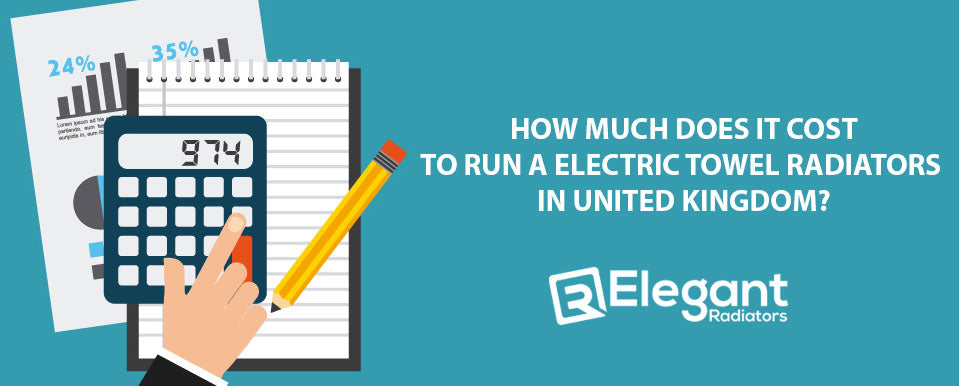# How Much Does It Cost To Run A Electric Towel Radiators in United Kingdom?You want to learn How Much Does It Cost To Run A Electric Towel Radiators in United Kingdom? Here we go.

In order to calculate the average operating cost for any electrical appliance you can use the following formula:

Watts/1000 = kW x Hours of operation = kW x kWh rate = Cost

Watts can usually be found on the appliance nameplate.

## Sample:  How much does it cost to operate my electric heater?

An electric heater wattage is usually given on the unit itself, or with the literature that comes with it. A simple example is 1000 watts.

To use this heater for 1 hour would cost based on UK standard tariff of 34.0p (September 2022) would be:

• Energy companies may have different electricity rates.

1000 watts = 1kW x1 hour of operation = 1 kWh x 34.0p = £0.34

This can then be calculated by hours used per day and by how many day although dependent on individual usage and of course the type of building it is being used in?

Is it double glazed, insulated, type of floor and roof etc. If we take are Arlec and use this calculation it will cost the following per hour which I have rounded up to give a true refection

Watt Calculate Per Hour
700 Watt 0.7 x 34.0p £0.09
900 Watt 0.9 x 34.0p £0.23
1250 Watt 1.25 x 34.0p £0.425
1500 Watt 1.5 x 34.0p £0.51
1800 Watt 1.8 x 34.0p  £0.612

I'm going to create a feature and benefit document that details the construction of the Arlec, how it works, and how much it costs to operate. If necessary, all sales employees may use this. For usage with clients and specifications, I'll also turn it into a power point presentation.

Using the same method, the operating expenses of our towel rail's standard and thermostatic components are as follows:

## Thermostatic

Watt Calculate Per Hour
300 Watt 0.3 x 34p £0.102
600 Watt 0.6 x 34.0p £0.204
900 Watt 0.9 x 34.0p

£0.306

## Standard Heating Element

Watt Calculate Per Hour
150 Watt 0.15 x 34.0p £0.051
250 Watt 0.25 x 34.0p £0.085
400 Watt 0.4 x 34.0p £0.136
600 Watt 0.6 x 34.0p £0.204
900 Watt 0.9 x 34.0p £0.306